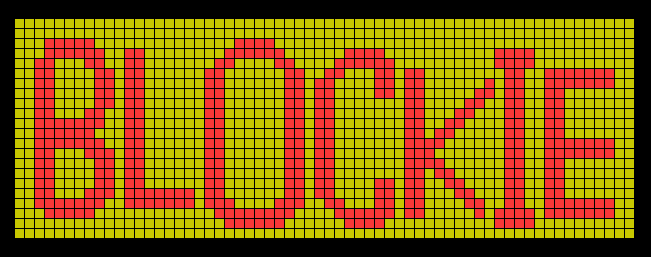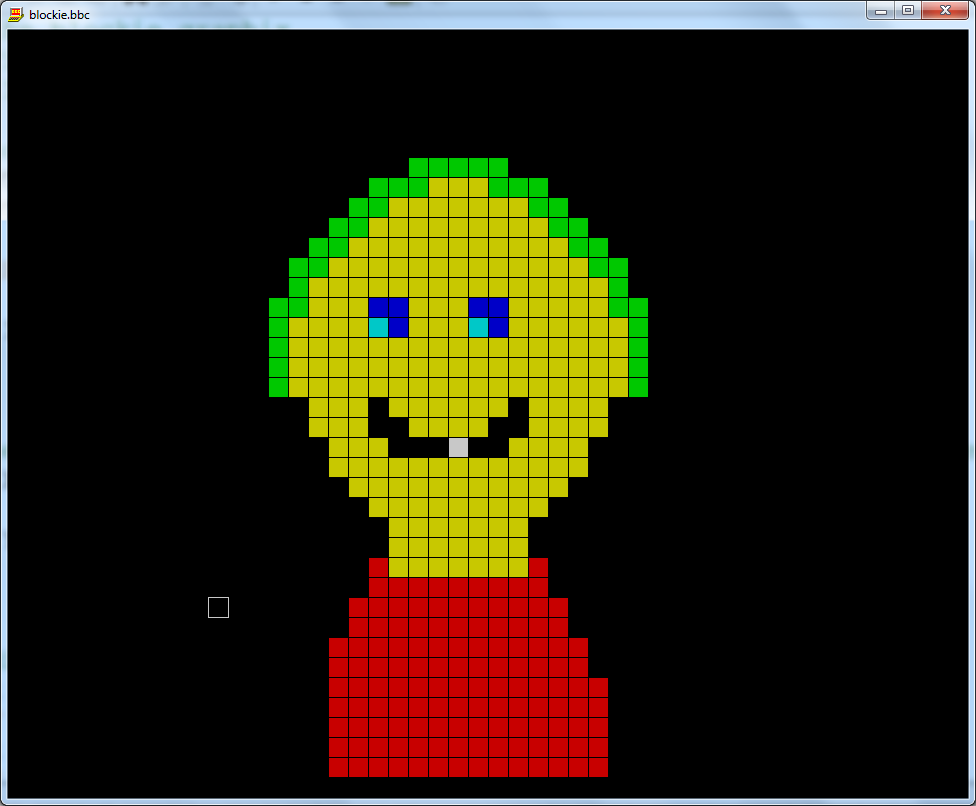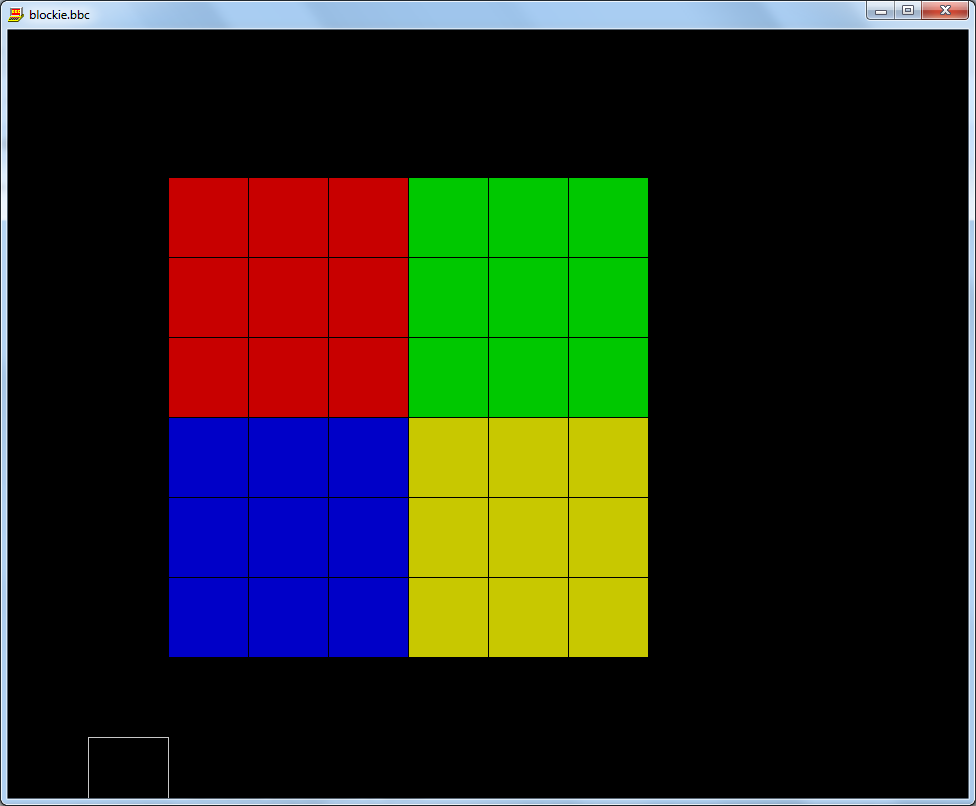## 12 days of Christmas

Happy Christmas everyone! I am sure you are all wondering how many presents you will get this year. This program will tell you how many presents you will get from your "true love" according to the song The Twelve Days of Christmas.

```       ```

`      repeat        print''"For how many days did your true love"        print"send you presents this christmas?"        input"> " n%               s% = ((n%/6)*(n%+2)*(n%+1))                print'"You got "str\$(s%)" present"+fn_plural(s%)+" in total"      until false                        deffn_plural(num%)      rem pass a number - num      rem returns "s" when num is not 1      local a\$      if num%=1 then        a\$ = ""      else        a\$ = "s"      endif      =a\$ `## Roman Numerals

A program for generating the first 3999 Roman numerals.

```       REM roman numerals      REM Tim Street      REM 12/11/2011                  PRINT "Enter an integer, between 1 and 3999 inclusive,"      PRINT "The program outputs the same number in Roman numerals."      REPEAT                REM get the user's number        REPEAT          ok% = TRUE : REM assume the best case          PRINT          INPUT "Enter an integer : " n%                    REM check boundaries          CASE TRUE OF            WHEN n%<=0              PRINT "Number entered is too low!"              ok% = FALSE                          WHEN n%> 3999              PRINT "Number entered is too high!"              ok% = FALSE                        ENDCASE          REM end of check boundaries        UNTIL ok%                REM the algorithm        WHILE n% >= 1000          PRINT "M";          n% -= 1000        ENDWHILE                IF n%>=900 THEN          PRINT "CM";          n% -= 900        ENDIF                WHILE n%>=500          PRINT "D";          n% -= 500        ENDWHILE                        WHILE n%>=400          PRINT "CD";          n% -= 400        ENDWHILE                        WHILE n% >= 100          PRINT "C";          n% -= 100        ENDWHILE                        IF n%>= 90 THEN          PRINT "XC";          n% -= 90        ENDIF                        WHILE n% >= 50          PRINT "L";          n% -= 50        ENDWHILE                        WHILE n% >= 40          PRINT "XL";          n% -= 40        ENDWHILE                        WHILE n% >= 10          PRINT "X";          n% -= 10        ENDWHILE                        WHILE n% >= 9          PRINT "IX";          n% -= 9        ENDWHILE                        WHILE n% >= 5          PRINT "V";          n% -= 5        ENDWHILE                        IF n% = 4 THEN          PRINT "IV"          n% = 0        ENDIF                        WHILE n% > 0          PRINT "I";          n% -= 1        ENDWHILE              UNTIL FALSE```## Blockie

This program allows you to paint coloured squares on the screen using the cursor keys.  It is essentially a very simple drawing program within a few lines of code.

There are options for choosing the size of the blocks and the colour.  There is loads of scope to change the program to make a more sophisticated drawing package - enjoy!`      `
`      REM the blockie graphix      REM Tim Goliath Street      REM 11/10/2012           REM screen parameters      X_WIDTH% = 1920      Y_WIDTH% = 1520           ON ERROR RUN           MODE 12  : OFF                     REM set the graphics cursor to somewhere in the middle of the screen      x% = X_WIDTH% DIV 2           y% = Y_WIDTH% DIV 2                     REM display instructions      *font Courier New, 40b      PRINT " BLOCKIE GRAPHIX"      *font Courier New, 12      PRINT "  By Mr Street"'      *font Courier New, 20b      PRINT " INSTRUCTIONS"      *font Courier New, 12      PRINT "  Use the CURSOR KEYS to move."      PRINT"  Press 1-9 for different colours"      PRINT"  Press SPACE for no colour"      PRINT"  Press DELETE to, erm... delete"      PRINT      PRINT"    (Press any key)"''      g% = GET           REPEAT        INPUT "  Enter block size (5-100) : " diam%      UNTIL diam%>=10 AND diam% <=100           diam% *= 2 : REM even numbers are best!      CLS                REM start with a random colour      currentCol% = RND(8)           REM MAIN LOOP      REPEAT        REM display the cursor        GCOL 7        RECTANGLE x%,y%,diam% , diam%               REM get user input        g% = GET               REM remove the cursor        GCOL 0        RECTANGLE x%,y%,diam% , diam%                      REM process user input        CASE g% OF                     WHEN 136            REM left                       IF x%>diam% THEN              x% -= diam%            ENDIF                     WHEN 137            REM right                       IF x%<X_WIDTH% THEN              x% += diam%            ENDIF                     WHEN 138            REM down            REM left            IF y%>0 THEN              y% -= diam%            ENDIF                     WHEN 139            REM up            REM right            IF y%<Y_WIDTH% THEN              y% += diam%            ENDIF                     WHEN 135, 8            REM delete, backspace            currentCol% = 0 : REM set to black                     WHEN 32            REM space            currentCol% = -1 : REM no colour                     WHEN 49,50,51,52,53,54,55,56,57,58            currentCol% = g%-48                   ENDCASE                      REM draw a rectangle in the current colour        IF currentCol% <> -1 THEN          GCOL currentCol%          RECTANGLE FILL x%, y%, diam% , diam%        ENDIF      UNTIL FALSE`